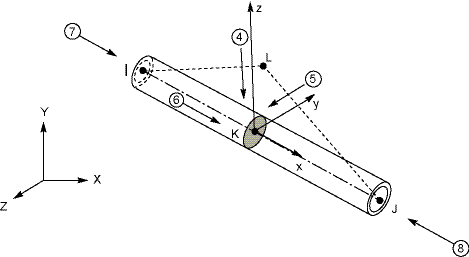## 13.289. PIPE289 - 3-D 3-Node PipeMatrix or Vector Shape Functions Integration Points
Stiffness and Stress Stiffness Matrices; and Thermal and Newton-Raphson Load Vectors Equation 11–19, Equation 11–20, Equation 11–21, Equation 11–22, Equation 11–23, and Equation 11–24

Along the length: 2

Across the section: see PIPE288 - 3-D 2-Node Pipe

Consistent Mass Matrix and Pressure, Hydrostatic, and Hydrodynamic Load VectorsSame as stiffness matrix

Along the length: 3 

Across the section: 1

Lumped Mass MatrixEquation 11–19, Equation 11–20, and Equation 11–21

Along the length: 3

Across the section: 1

1. For partially submerged elements, the hydrostatic/hydrodynamic load vector integration point(s) is/are centered on the wetted portion.

Element Temperature

KEYOPT(1) = 0 Linear thru wall and linear along length

KEYOPT(1) = 1 Bilinear across cross-section and linear along length

Nodal Temperature Constant across cross-section, linear along length
Internal and External Pressures Constant, except as adjusted by the affect of internal and external fluids.

References: Simo and Vu-Quoc(), Ibrahimbegovic().

The theory for this element is identical to that of PIPE288 - 3-D 2-Node Pipe, except that this element is a 3-node pipe.